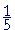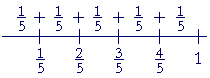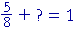S k i l l
i n
A R I T H M E T I C

Lesson 21

# UNIT FRACTIONS

A unit -- "one" -- is the source of a number of anything.
We count units.

 1. What is a unit fraction? A fraction with 1 as its numerator.Each unit fraction is a part of number 1.  Half of 1, a third, a fourth, and so on.

Here is how we count's. "One fifth, two fifths, three fifths," and so on.

Every fraction is thus a number of unit fractions.In the fraction 35 , the unit is 15 .  And there are 3 of them.
 35 = 15 + 15 + 15 .

The denominator of a fraction names the unit  The numerator tells their number -- how many.

Example 1.   In the fraction, what number is the unit, and how many of them are there?

 Answer.   The unit is 16 .  And there are 5 of them.
 56 = 5 × 16 = 16 + 16 + 16 + 16 + 16 .
 Example 2.   Let 13 be the unit -- and count to 2 13 .Again, every fraction is a sum -- a number -- of unit fractions.

 23 =  2 × 13 = 13 + 13 .
 38 =  3 × 18 = 18 + 18 + 18 .

The symbols for all the numbers of arithmetic
stand for a sum of units.

 Example 3.   Add 28 + 38 .
 Answer. 58 .
 2 eighths + 3 eighths are 5 eighths. The unit we are adding is 18 .

This illustrates the following principle:

We can only add or subtract things that have the same name,
which we call the unit.
In the Example above , the name of what we are adding is "Eighths."

We will see this in Lesson 25.  The denominator of a fraction has no other function but to name the unit.

 59 − 39 = 29 .

Example 4.   1 is how many fifths?

 Answer.   1 = 55 ("Five fifths.")15 is contained in 1 five times.

Similarly,

 1 = 33 = 44 = 1010

And so on.  We may express 1 as a fraction with any denominator.

 Example 5.   Add, and express the sum as an improper fraction: 59 + 1.
 Answer. 59 + 1 = 59 + 99 = 14 9 .

It was necessary to express 1 as so many ninths because the things we add must have the same name.2. How can we express a whole number as fraction with a given denominator?Multiply the denominator by the whole number. Make that product the numerator.

 Example 8.    2 = 2 × 5   5 = 10 5 .
 Since 1 = 55 , then 2 is twice as many fifths:  2 = 10 5 .   3 = 15 5 .   4 = 20 5 .

And so on.

 Example 9.    6 = ?3
 Answer.    6  = 6 × 3   3 = 18 3 .
 Example 10.     How many times is 18 contained in 5?  That is, 5 = ?8 .
 Answer.   5  = 40 8 .
 Example 11.    Add: 53 + 4.
 Answer. 53 + 4 = 53 + 12 3 = 17 3 = 5 23 .

Let us now revisit the rule for changing a mixed number to an improper fraction (Lesson 20).  In fact, we will see why we have that rule.

 3. How do we change a mixed number to an improper fraction?Change the whole number to a fraction with the same denominator. Then add those fractions.
 Example 12.   3 58 = 3 + 58 = 24 8 + 58 = 29 8 .
 Example 13.   5 27 = 5 + 27 = 35 7 + 27 = 37 7 .

The complement of a proper fraction

 4. What do we mean by the complement of a proper fraction?It is the proper fraction we must add in order to get 1.
 Example 14. 58 + ? = 1
 Answer.   Since 1 = 88 , then 58 + 38 = 1
 38 is called the complement of 58 . 38 completes 58 to make 1.

Equivalently, since finding what number to add  is subtraction:

 1 − 58 = 38 .
 Example 15.    1 − 35 = 25 .
 When we add 25 to 35 ,  we get 55 , which is 1.
 25 is the complement of 35 .

Example 16.   Compare

 1 − 14 and  6 − 14 .
 First, since 1 is 44 , then
 1 − 14 = 34 ,
 which is the complement of 14 .

Look:since we are subtracting 14 -- which is less than 1 -- from 6, the answer
 will fall beween 5 and 6.  It will be 5 34 .  Again, 34 is the complement of 14 .

In other words:

 5. What will be the answer when we subtract a proper fraction from a whole number greater than 1?It will be a mixed number which is one whole number less, and whose fraction is the complement of the proper fraction.
 Example 17. 5 − 13 = 4 234 is one less than 5.  And 23 is the complement of 13 .
 In fact, whenever we subtract 13 from a whole number, the fractional
 part will be 23 .
 12 − 13 = 11 23 . 25 − 13 = 24 23 . 38 − 13 = 37 23 .

And so on.

 Example 18.   9 − 25 =  8 35 .

We could even check that by adding:

 8 35 + 25 = 8 55 = 9.

At this point, please "turn" the page and do some Problems.

or

Continue on to the next Lesson.

Copyright © 2019 Lawrence Spector

Questions or comments?

E-mail:  themathpage@yandex.com

Private tutoring available.